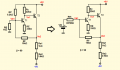# Transistor circuit

#### ericgibbs

Joined Jan 29, 2010
9,537

#### Zeeus

Joined Apr 17, 2019
508
I did this oo..even used the spice file you provided with the My_3904 but yet can't see

But i give up..so many frustration for one night : go to bed

#### ericgibbs

Joined Jan 29, 2010
9,537
OK, get some sleep, tomorrow is a new day, we can then continue.E

•Zeeus

#### Bordodynov

Joined May 20, 2015
2,497

#### Audioguru again

Joined Oct 21, 2019
867
They cannot make a 2N3904 or any other transistor with a certain beta. Some that are made on a Friday have a gain of only 99 and some that are made on a Tuesday have a gain of 300 or more.
But a simulator program don't no dat.

#### Jony130

Joined Feb 17, 2009
5,145
If you want to find the DC operating point.
We start by assuming β = ∞ and apply a Thevenin theorem to RP and RB.

Vth = 5V*1kΩ/(6.2kΩ + 1kΩ) = 0.694V and Rth = RP||RB = 0.861kΩ

And the situation will look like this:Therefore from KVL we can write:

Vcc - Ic1*Rc - Vbe2 - Ix*RF - Vbe1- Ic1*RE = 0 (1)
Vcc - Ic1*Rc - Vbe2 - Ix*(RF + Rth) - Vth = 0 (2)

And we can solve this for Ic1 and Ix

$I_C = \frac{R_F (V_{TH} - V_{BE}) + R_{TH}*(V_{CC} - 2 V_{BE})}{R_C R_{TH} + R_E (R_F + R_{TH})} \approx 187\mu A$

And if we know Ic1 we can find all other voltages and current in the circuit.

Ie2 ≈ ((Vcc - Ic1*Rc - Vbe) - Vee)/(Re1 + Re2) ≈ (0.286V - ( -5V))/4.9kΩ ≈ 1mA

Also, this circuit is very Vbe sensitive, changing the Vbe to 0.7V changes the current very much Ic1 ≈ 135μA and Ie2 ≈ 1.3mA.
Therefore exact calculations are redundant due to the fact that we do not know the exact value of a Vbe and Vbe mismatch, and the same we can say about beta value.

#### Zeeus

Joined Apr 17, 2019
508
If you want to find the DC operating point.
We start by assuming β = ∞ and apply a Thevenin theorem to RP and RB.

Vth = 5V*1kΩ/(6.2kΩ + 1kΩ) = 0.694V and Rth = RP||RB = 0.861kΩ

And the situation will look like this:
View attachment 194161

Therefore from KVL we can write:

Vcc - Ic1*Rc - Vbe2 - Ix*RF - Vbe1- Ic1*RE = 0 (1)
Vcc - Ic1*Rc - Vbe2 - Ix*(RF + Rth) - Vth = 0 (2)

And we can solve this for Ic1 and Ix

$I_C = \frac{R_F (V_{TH} - V_{BE}) + R_{TH}*(V_{CC} - 2 V_{BE})}{R_C R_{TH} + R_E (R_F + R_{TH})} \approx 187\mu A$

And if we know Ic1 we can find all other voltages and current in the circuit.

Ie2 ≈ ((Vcc - Ic1*Rc - Vbe) - Vee)/(Re1 + Re2) ≈ (0.286V - ( -5V))/4.9kΩ ≈ 1mA

Also, this circuit is very Vbe sensitive, changing the Vbe to 0.7V changes the current very much Ic1 ≈ 135μA and Ie2 ≈ 1.3mA.
Therefore exact calculations are redundant due to the fact that we do not know the exact value of a Vbe and Vbe mismatch, and the same we can say about beta value.
Also did thevenin like you did but notice Vth = 0.694 but the VB1 is greater than this.. it's 754mv

#### Jony130

Joined Feb 17, 2009
5,145
So what? Vth is not the voltage at the T1 base.

•Zeeus

#### Jony130

Joined Feb 17, 2009
5,145
For Vbe = 0.6V we have Ic1 ≈ 187μA and VB1 ≈ 187μA*330Ω + 0.6V ≈ 0.662V

And for Vbe = 0.7V ---> Ic1 ≈ 135μA ---> VB1 ≈ 135μA*330Ω + 0.7V ≈ 0.745V

All this assuming Ib1 = Ib2 = 0A

#### Zeeus

Joined Apr 17, 2019
508
So what? Vth is not the voltage at the T1 base.
Thought the voltage will be less than Vth but see my mistake now

#### Zeeus

Joined Apr 17, 2019
508
If you want to find the DC operating point.
We start by assuming β = ∞ and apply a Thevenin theorem to RP and RB.

Vth = 5V*1kΩ/(6.2kΩ + 1kΩ) = 0.694V and Rth = RP||RB = 0.861kΩ

And the situation will look like this:
View attachment 194161

Therefore from KVL we can write:

Vcc - Ic1*Rc - Vbe2 - Ix*RF - Vbe1- Ic1*RE = 0 (1)
Vcc - Ic1*Rc - Vbe2 - Ix*(RF + Rth) - Vth = 0 (2)

And we can solve this for Ic1 and Ix

$I_C = \frac{R_F (V_{TH} - V_{BE}) + R_{TH}*(V_{CC} - 2 V_{BE})}{R_C R_{TH} + R_E (R_F + R_{TH})} \approx 187\mu A$

And if we know Ic1 we can find all other voltages and current in the circuit.

Ie2 ≈ ((Vcc - Ic1*Rc - Vbe) - Vee)/(Re1 + Re2) ≈ (0.286V - ( -5V))/4.9kΩ ≈ 1mA

Also, this circuit is very Vbe sensitive, changing the Vbe to 0.7V changes the current very much Ic1 ≈ 135μA and Ie2 ≈ 1.3mA.
Therefore exact calculations are redundant due to the fact that we do not know the exact value of a Vbe and Vbe mismatch, and the same we can say about beta value.
Thanks so much for helping with insight but solution is 6/10 but thanks. Ib = 0...